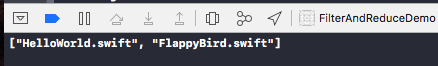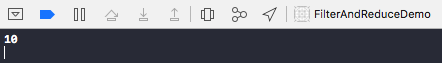# 高阶函数---swift中的Filter 和 Reduce# Filter

``````let exampleFiles = ["README.md", "HelloWorld.swift", "FlappyBird.swift"]
``````

``````func getSwiftFiles(_ files: [String]) -> [String] {
var result: [String] = []
for file in files {
if file.hasSuffix(".swift") {
result.append(file)
}
}
return result
}
``````

``````print("\(getSwiftFiles(exampleFiles))")

````````````extension Array {
func filter(includeElement: (Element) -> Bool) -> [Element] {
var result: [Element] = []
for x in self where includeElement(x) {
result.append(x)
}
return result
}
}
``````

``````func getSwiftFiles(_ files: [String]) -> [String] {
return files.filter(includeElement: { (file) -> Bool in
file.hasSuffix(".swift")
})
}
``````

# Reduce

``````func sum(_ xs: [Int]) -> Int {
var result: Int = 0
for x in xs {
result += x
}
return result
}
``````

``````print(sum([1, 2, 3, 4]))
````````````func product(_ xs: [Int]) -> Int {
var result: Int = 1
for x in xs {
result = x * result
}
return result
}
``````

``````func concatenate(_ xs: [String]) -> String {
var result: String = ""
for x in xs {
result += x
}
return result
}
``````

``````func prettyPrintArray(_ xs: [String]) -> String {
var result: String = ""
for x in xs {
result = " " + result + x + "\n"
}
return result
}
``````

``````extension Array {
func reduce<T>(initial: T, combine: (T, Element) -> T) -> T {
var result = initial
for x in self {
result = combine(result, x)
}
return result
}
}
``````

``````func sumUsingReduce(_ xs: [Int]) -> Int {
return xs.reduce(0) { (result, x) -> Int in
return result + x
}
}
``````

``````func productUsingReduce(_ xs: [Int]) -> Int {
return xs.reduce(initial: 1, combine: *)
}

func concatUsingReduce(_ xs: [String]) -> String {
return xs.reduce(initial: "", combine: +)
}
``````

``````func flatten<T>(_ xss: [[T]]) -> [T] {
var result: [T] = []
for xs in xss {
result += xs
}
return result
}
``````

``````func flattenUsingReduce<T>(_ xss: [[T]]) -> [T] {
return xss.reduce(initial: [], combine: { (result, xs) -> [T] in
return result + xs
})
}
``````

``````func mapUsingReduce<T>(_ transform: (Element) -> T) -> [T] {
return reduce(initial: [], combine: { (result, x) -> [T] in
return result + [transform(x)]
})
}

func flterUsingReduce(_ includeElement:(Element) -> Bool) -> [Element] {
return reduce(initial: [], combine: { (result, x) -> [Element] in
return includeElement(x) ? result + [x] : result
})
}
``````